# Linear Codes

Linear codes provide an optimal way for transmitting blocks of data over noisy channels

Originator: Andries E. Brouwer and Markus Grassl

## Examples

### Basic Examples

Retrieve the resource:

 In:=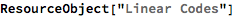Out=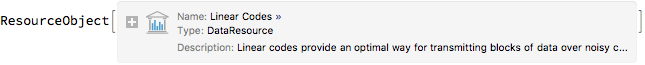Retrieve the default content:

 In:=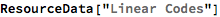Out=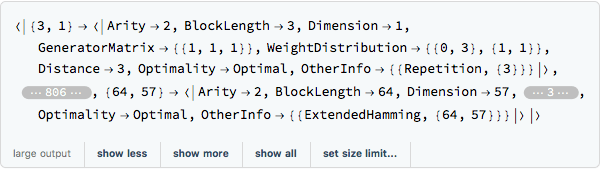### Other Examples

Show data for the Golay code (A further exploration can be found at the Wolfram Demonstration Golay Code):

 In:=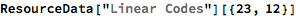Out=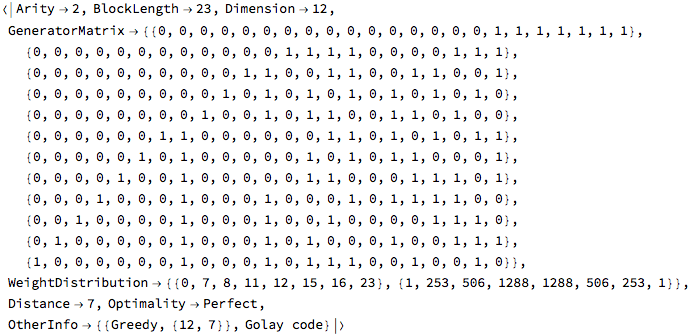Generate a triangle of optimal distances with the blocklength (length of the codeword) on the left and the dimension (number of data bits) on the bottom:

 In:=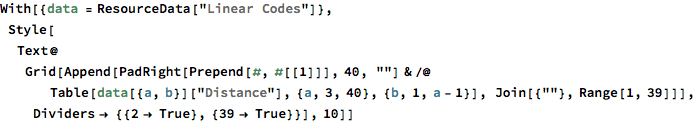Out=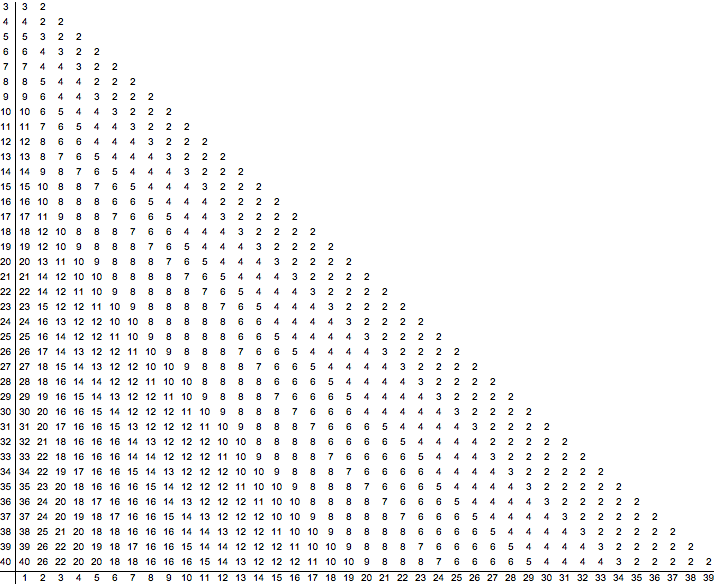Generate a grid of some of the optimal generator matrices:

 In:=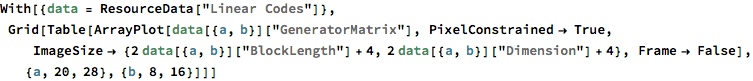Out=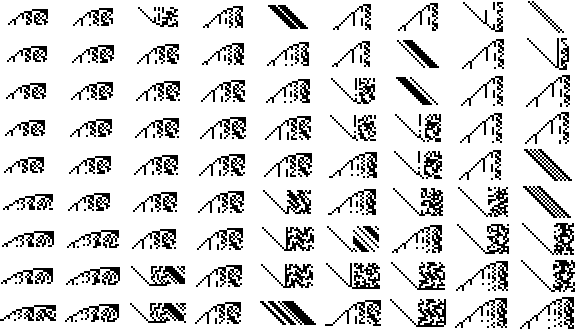Wolfram Research, "Linear Codes" from the Wolfram Data Repository (2017) https://doi.org/10.24097/wolfram.23530.data